Chapter Notes: Some Applications of Trigonometry

# Some Applications of Trigonometry Chapter Notes - Mathematics (Maths) Class 10

 Table of contentsHeight and DistancesLine of SightAngle of ElevationAngle of DepressionSolved ExamplesTrigonometry, the branch of mathematics dealing with the relationships between the angles and sides of triangles, has several practical applications in various fields.
This chapter discusses the practical applications of trigonometry in everyday life. In trigonometry, we  measure sides of triangle, when particular angle is given.

## Height and Distances

Most the buildings, walls, towers we see around are perpendicular to ground. This chapter deals with measurement of heights and distance from certain points with the help of trigonometry if particular angles are known.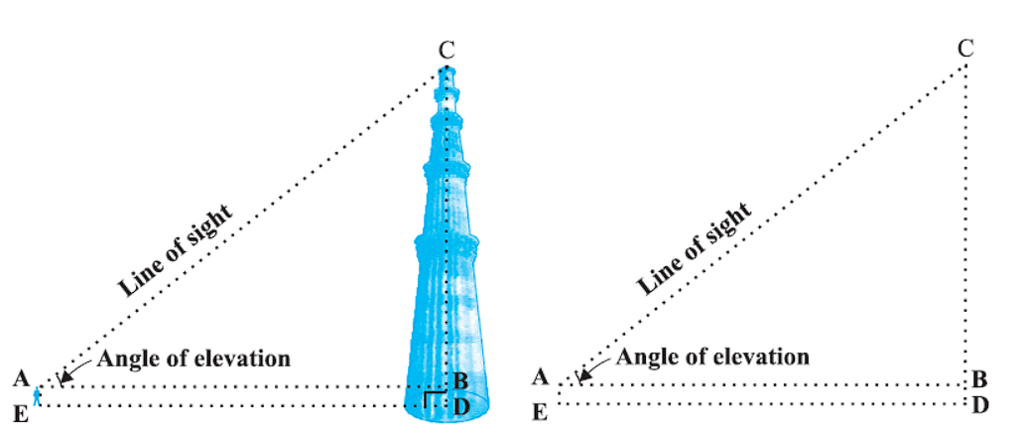• In the diagram, the line AC from the student's eye to the top of a minar is the line of sight. The angle BAC between the line of sight and the horizontal is the angle of elevation.
• The line of sight is drawn from an observer's eye to the object they're looking at.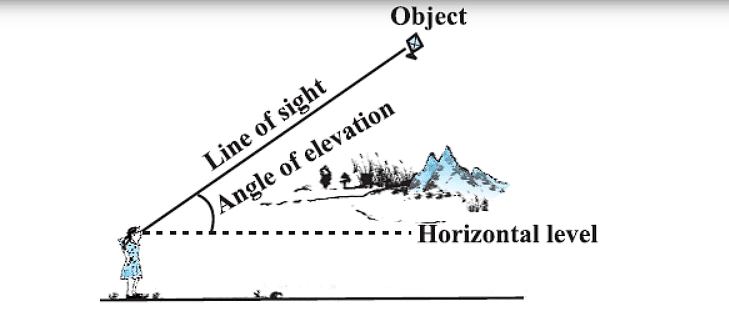• Angle of elevation is the angle between the line of sight and the horizontal when viewing an object above eye level.

The line of sight here is below the horizontal, forming the angle of depression. Angle of depression is the angle between the line of sight and the horizontal when viewing an object below eye level.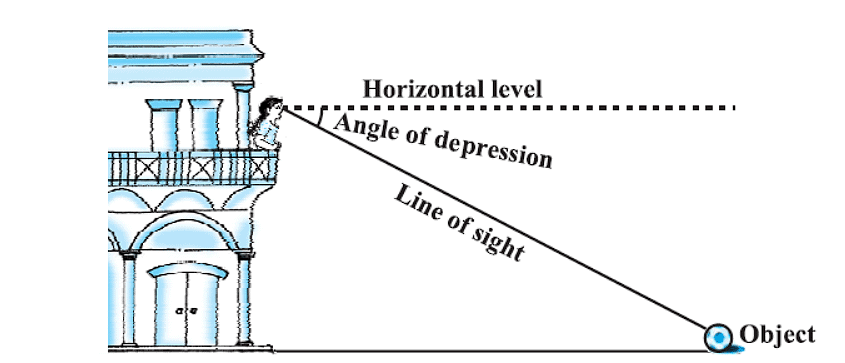## Line of Sight

When an observer looks from a point O at an object A, then OA is called line of sight.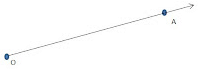## Angle of Elevation

Suppose that from a point O, you look up at point A, then the angle, the which the line of sight makes with the horizontal line through O is called the angle of elevation of A, as we seen from O.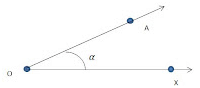Here OX is horizontal line. is here angle of elevation.

## Angle of Depression

Suppose that from the point O, you look down at an object B, placed below the level of your eye. Then the angle which the line of sight makes with the horizontal line through O is called angle of depression of B for O.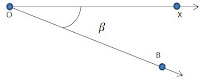Here, OX is horizontal line. β is here angle of deviation.

### An Example of Angle of Elevation and Depression

A man standing on a building will look at a building higher than his. If he sees at the top, he will look at the top he will form angle of elevation. If he look at the bottom he will form angle of depression with bottom of the building.

## Solved Examples

Q1: The angle of elevation of a cloud 60m above a lake is 30° and the angle of reflection of the cloud in the lake is 60°. Find height of the cloud.

Sol: The situation is depicted by the following diagram.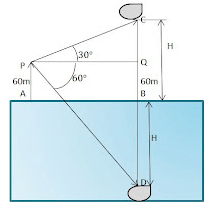Let AB be the surface of the lake and let P be the point vertically above A such that AP=60m

Let C be the position of cloud and let D be its reflection in the lake.

Let the height of Cloud be H meters.

Then BC=BD=H (Let)

Draw PQ Perpendicular to CD

⇒ ∠QPC=30°, ∠QPD=60°

BQ=AP=60m

CQ=(H-60)m, DQ=(H+60)m

From right triangle ∆ CQP, we have
Let AB be the surface of the lake and let P be the point vertically above A such that AP=60m
Let C be the position of cloud and let D be its reflection in the lake.
Let the height of Cloud be H meters.

Then BC=BD=H (Let)
Draw PQ Perpendicular to CD
⇒∠QPC=30°,∠QPD=60°
BQ=AP=60m
CQ=(H-60)m, DQ=(H+60)m

From right triangle ∆ CQP, we have
PQ/CQ=cot⁡30°
PQ/(H-60) = √3
PQ⇒(H-60) √3 m (i)

From the right triangle ∆ DQP
PQ/DQ=cot⁡60°
⇒PQ=(H+60)/√3 (ii)

From (i) and (ii), we get
(H-60) √3=(H+60)/√3
⇒3H-180=H+60
⇒H=240
So, height of the cloud is H=240m.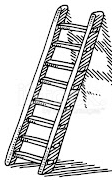The angle of elevation of a ladder leaning against a wall is 45° and foot of the ladder is 10m away from the wall. Find the length of ladder. The following diagram depicts the situation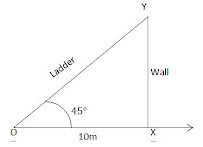Let OY is the ladder. Let OY=l. YX is the wall on which ladder is leaning on. It is given, ladder is leaned at 45°. Also, distance from the wall to foot of ladder is 10m, OX=d=10m. It is given √2=1.414
From the diagram,
cos 45°= OX/OY = d/l
⇒ l=d/cos 45°
We know that cos 45° = 1/√2
So, l = d/(1/√2) = √2 d
l = √2×10 m = 10√2 m = 10×1.414 = 14.14m
So, length of ladder is 14.14m

The document Some Applications of Trigonometry Chapter Notes | Mathematics (Maths) Class 10 is a part of the Class 10 Course Mathematics (Maths) Class 10.
All you need of Class 10 at this link: Class 10

## Mathematics (Maths) Class 10

115 videos|478 docs|129 tests

## FAQs on Some Applications of Trigonometry Chapter Notes - Mathematics (Maths) Class 10

 1. What are some real-life examples of the application of trigonometry?Ans. Trigonometry is widely used in various fields. Some real-life examples of its application include calculating the height of a building or a mountain using angles and distances, determining the distance between two points using trigonometric ratios, and predicting the position of celestial bodies in astronomy using trigonometric functions.
 2. How is trigonometry useful in navigation and surveying?Ans. Trigonometry plays a crucial role in navigation and surveying. It helps in determining the angles and distances between various points, which are essential for creating accurate maps, measuring land areas, and planning routes for navigation. Trigonometric functions are used to calculate bearings, azimuths, and vertical angles, making it easier to navigate and survey accurately.
 3. How does trigonometry help in solving problems related to triangles?Ans. Trigonometry is specifically designed to solve problems related to triangles. It provides various trigonometric ratios such as sine, cosine, and tangent, which help in finding unknown sides and angles of a triangle. By using these ratios, one can determine the length of a side or measure an angle of a triangle without directly measuring them.
 4. Can trigonometry be used to measure the height of a tall object?Ans. Yes, trigonometry can be used to measure the height of a tall object. By measuring the distance from the object and the angle of elevation or depression, one can use trigonometric functions to calculate the height. This method is commonly used in fields like engineering, architecture, and construction to estimate the height of buildings, towers, and other structures.
 5. How is trigonometry applied in the field of physics?Ans. Trigonometry is extensively used in physics to analyze and solve problems related to motion, forces, and waves. It helps in calculating distances, velocities, and accelerations by utilizing trigonometric functions. For example, projectile motion can be analyzed using trigonometry to determine the range, maximum height, and time of flight of a projectile. Additionally, trigonometry is used in analyzing the behavior of waves and calculating wave frequencies, wavelengths, and amplitudes.

## Mathematics (Maths) Class 10

115 videos|478 docs|129 testsExplore Courses for Class 10 examSignup to see your scores go up within 7 days! Learn & Practice with 1000+ FREE Notes, Videos & Tests.
10M+ students study on EduRev
Track your progress, build streaks, highlight & save important lessons and more!
Related Searches

,

,

,

,

,

,

,

,

,

,

,

,

,

,

,

,

,

,

,

,

,

;